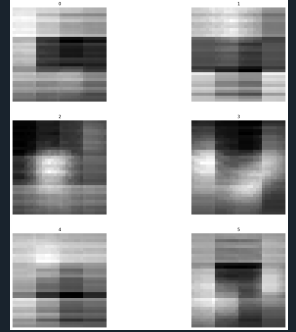# CVAE on CIFAR10 Dataset

Hi. I was running the code from the following repository.(CVAE_MNIST/train_cvae.py at master · debtanu177/CVAE_MNIST · GitHub)
I was wondering if there was a way to adapt this to run on the CIFAR10 Dataset. Since the images are 32x32 instead of 28x28, would anyone be able to suggest how to change the structure of the convolutional and fully connected layers of the model and also the encoder and decoder, as well as any other parts of the Model which would need to be adjusted? I would appreciate any help on how to do this. Thanks.

You would need to change a few feature shapes etc. in the model and also change the processing of the label tensor which is concatenated to the input.
This model should work for CIFAR10:

``````class Model(nn.Module):
def __init__(self,latent_size=32,num_classes=10):
super(Model,self).__init__()
self.latent_size = latent_size
self.num_classes = num_classes

# For encode
self.conv1 = nn.Conv2d(3+1, 16, kernel_size=5, stride=2)
self.conv2 = nn.Conv2d(16, 32, kernel_size=5, stride=2)
self.linear1 = nn.Linear(5*5*32,300)
self.mu = nn.Linear(300, self.latent_size)
self.logvar = nn.Linear(300, self.latent_size)

# For decoder
self.linear2 = nn.Linear(self.latent_size + self.num_classes, 300)
self.linear3 = nn.Linear(300,4*4*32)
self.conv3 = nn.ConvTranspose2d(32, 16, kernel_size=5,stride=2)
self.conv4 = nn.ConvTranspose2d(16, 1, kernel_size=5, stride=2)
self.conv5 = nn.ConvTranspose2d(1, 1, kernel_size=8)

def encoder(self,x,y):
y = torch.argmax(y, dim=1).reshape((y.shape,1,1,1))
y = y.expand(-1, -1, x.size(2), x.size(3))
t = torch.cat((x,y),dim=1)

t = F.relu(self.conv1(t))
t = F.relu(self.conv2(t))
t = t.reshape((x.shape, -1))
print(t.shape)

t = F.relu(self.linear1(t))
mu = self.mu(t)
logvar = self.logvar(t)
return mu, logvar

def reparameterize(self, mu, logvar):
std = torch.exp(0.5*logvar)
eps = torch.randn_like(std).to(device)
return eps*std + mu

def unFlatten(self, x):
return x.reshape((x.shape, 32, 4, 4))

def decoder(self, z):
t = F.relu(self.linear2(z))
t = F.relu(self.linear3(t))
t = self.unFlatten(t)
t = F.relu(self.conv3(t))
t = F.relu(self.conv4(t))
t = F.relu(self.conv5(t))
return t

def forward(self, x, y):
mu, logvar = self.encoder(x,y)
z = self.reparameterize(mu,logvar)

# Class conditioning
z = torch.cat((z, y.float()), dim=1)
pred = self.decoder(z)
return pred, mu, logvar
``````

Thanks so much for the help, as it runs now! However, the loss is very high and the images are quite blurry, could that be because there is too much noise in the image, and would you have any suggestions on how I could try to prevent this? Also, the images that are being generated are black and white but CIFAR10 is RGB, so would there be any changes I could make so the images are RGB? Thanks!This is what the output looks like after 17 epochs.

You could use 3 output channels in `self.conv5` which could then be interpreted as RGB channels.

Thanks for the reply, I changed the line to self.conv5 = nn.ConvTranspose2d(1, 3, kernel_size=8)
but the output is still Black and White. Also do you have any idea as to how I could improve the model since the loss is very high (around 9000) even after 100 epochs? Thank you so much for your help!

You might need to change the colormap of your plot library to be able to see color outputs or the current output contains the same values of all 3 channels, which would then also be grayscale.

No, I don’t know what issue you might be hitting during the training of the CVAE.

Thanks for the help, it now shows color outputs.